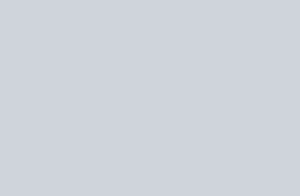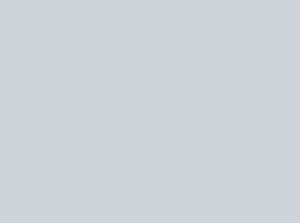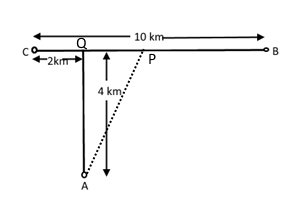Stay Update with us

Email Subscription for Get Latest Notification and MCQ Quiz

# CTET 2011 Question Paper-2 with Answer

## CTET 2011 Question Paper-2 with Answer

Child DevelopmentMathematics and ScienceSocial studiesLanguage-I(Eng)Language-II(Hindi)

Candidate has to do questions number 31 to 90 either from Part-II ( Mathematics and Science ) of from Part-III ( Social Studies/ Social Science).

#### Mathematics and Science

Directions: (Q. 31-90) Answer the following questions by selecting the most appropriate option.

Q31. The ratio between the length and perimeter of a rectangular plot is 1:3. What is the ratio between the length and breadth of the plot?

(a) 1 : 2

(b) 2:1

(c) 3:2

 Answer: (b) 2:1Sol: Let length and breadth of rectangular are x and y∴ perimeter = 2(x + y)According to question,$\frac{a}{2\left( a+b \right)}=\frac{1}{3}$ $\Rightarrow \,\,3a\,\,=\,\,2a\,\,+\,\,2b$ $\Rightarrow a\,\,=\,\,2b$ $\Rightarrow \frac{a}{b}=\text{2:}1$

Q32. If a x b = a2 + b2 and a. b= a2 – b2, the value of (5 x 2).25 is

(a) 215

(b) 225

(c) 226

(d) 216

 Answer: (d) 216Solution: 5 x 2 = 25 + 4 = 29Now (5 x 2).25 = 29.25 = 292 – 252 = (29 + 25)(29 – 25)=216

Q33. If a, b and c are three natural numbers in ascending order, then

(a) c2 – a2 > b

(b) c2 – a2 =  b2

(c) c2 – a2 <  b2

(d) c2 + a2 =  a2

 Answer: (a) c2 – a2 > bSolution: we have a < b < cNow, c2 – a2 = (c – a) (c + a), as c > b then c + a > b and a, b, c natural number then c – a will minimum be 2.Hence c2 – a2 > b

Q34. Buy three, get one free.’ What is the percentage of discount being offered here?

(a) 33.33%

(b) 25%

(c) 20%

(d) 28.56%

 Answer: (b) 25%Solution: Buy three, get one free means discount one out of 4 items,∴ discount = 1/4 × 100 = 25%

Q35. The value is $\sqrt{2}\,\,+\,\,\sqrt{3}\,\,+\,\,\sqrt{2}\,\,-\,\,\sqrt{3}$

$\left( a \right) \sqrt{6}$

$\left( b \right) \,\,6$

$\left( c \right) 2\sqrt{2}$

$\left( c \right) 2\sqrt{3}$

 Answer: $\left( c \right) 2\sqrt{2}$Solution: $\sqrt{2}\,\,+\,\,\sqrt{3}\,\,+\,\,\sqrt{2}\,\,-\,\,\sqrt{3}=2\sqrt{2}$

Q36. On recast, the radius of an iron rod is made one fourth. If its volume remains constant, then the new length will become

(a) 1/4 times of the original

(b) 1/16 times of the original

(c) 16 times of the original

(d) 4 times of the original

 Answer: (c) 16 times of the originalSolution: let radius and length are r and lNow volume V = ∏r2lAfter change radius and length are r1=r/4 and l1volume V1 = ∏ (r/4)2 l1Now,  ∏r2l = ∏ (r/4)2 l1⇒ l = l1/16⇒ l1 = 16l∴ 16 times of the original

Q37. Find the value of 547527/82 if 547.527/0.0082 = x.

(a) x/10

(b) 10

(c) 100x

(d) x/100

 Answer: (a) x/10Solution: $\frac{547.527}{0.0082}=x\,\,\Rightarrow \,\,\frac{547.527\times 10000}{0.0082\times 10000}=x\,\,\Rightarrow \frac{547527\times 10}{82}=x\,\,\Rightarrow \frac{547527}{82}=\frac{x}{10}$

Q38. The smallest number by which 68600 must be multiplied to get a perfect cube is

(a) 5

(b) 10

(c) 8

(d) 12

 Answer: (a) 5Solution: 68600 = 2 × 2 × 2 × 5 × 5 × 7 × 7 × 7 = ( 2 × 7)3 × 5 × 5∴ it should be multiplied by 5 to make perfect cube.

Q39. A cyclist at ‘C’ is cycling towards B. How far will he have to cycle from C before he is equidistant from both A and B?(b) 3 km

(c) 6 km

(d) 5 km

 Answer: (d) 5 kmSolution:Let the cyclist x km from point C towards B at P point where he is equidistant from both A and B.CP = x km, PQ =( x – 2) km, PB = (10 – x) kmNow, AQ2 + PQ2 = AP2 = PB2⇒ 16 + (x–2)2 = (10 – x)2⇒ 16 + x2 – 4x + 4 = 100 –20x + x2⇒ 16x = 80⇒ x = 5

Q40. Unit’s digit in 132003 is

(a) 1

(b) 3

(c) 7

(d) 9

 Answer: (c) 7Solution: Remainder of 2003/4 = 3, now 33 = 27Then unit digit in 132003  is 7

Pages ( 4 of 20 ): « Previous123 4 56 ... 20Next »

### Quiz-5: Ancient Indian History MCQ Questions with Answer

error: Content is protected !!